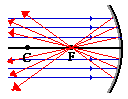Mirror

Plane Mirror

A flat mirror that reflects light rays in the same order as they approach the mirror.Concave Mirror

A converging mirror; light rays that strike the mirror surface are reflected so that they converge, or "come together," at a pointConvex Mirror

A diverging mirror; light rays that strike the mirror surface are reflected so that they diverge, or "go apart," and they never come to a point.Definition Provided by Peggy Schweiger

Mirror Vocabulary

Ray Tracing:  In physics, ray tracing is a method for calculating the path light is travel through a system with regions of varying propagation velocity, absorption characteristics, and reflecting surfaces. Under these circumstances, wave fronts may bend, change direction, or reflect off surfaces. Ray tracing solves this problem by idealizing light as narrow beams called rays through the medium by discrete amounts. Simple problems can be analyzed by propagating a few rays using simple mathematics.

Definition provided by Wikipedia

Real Image:  Real image is a point of convergence of the rays of light that make up the image. If a screen is placed in the plane of a real image the image will generally become visible and clear on the screen. Examples of real images include the image seen on a cinema screen (the source being the projector), the image produced on a detector in the rear of a camera, and the image produced on an eyeball retina (the camera and eye focus light through an internal convex lens). In ray diagrams (such as the images on the right), real rays of light are always represented by full, solid lines; perceived or extrapolated rays of light are represented by dashed lines. A real image occurs where rays converge, whereas a virtual image occurs where rays only appear to converge.

Definition provided by Wikipedia

Virtual image:  virtual image is an image in which the outgoing rays from a point on the object always diverge. It will appear to converge in or behind the optical device (i.e., a mirror). A simple example is a flat mirror where the image of oneself is perceived at twice the distance from oneself to the mirror. That is, if one is half a meter in front of the mirror, one's image will appear to be at a distance of 1 meter away (or half a meter inside or behind the mirror). Because the rays never really converge, one cannot project a virtual image.

By contrast, a real image is an image in which the outgoing rays from a point on the object pass through a single point. It is easiest to observe real images when projected on an opaque screen. A screen is not necessary for the image to form.

Definition provided by Wikipedia

Converging and Diverging Mirrors:   If a concave mirror were thought of as being a slice of a sphere, then there would be a line passing through the center of the sphere and attaching to the mirror in the exact center of the mirror. This line is known as the principal axis. The point in the center of the sphere from which the mirror was sliced is known as the center of curvature and is denoted by the letter C in the diagram below. The point on the mirror's surface where the principal axis meets the mirror is known as the vertex and is denoted by the letter A in the diagram below. The vertex is the geometric center of the mirror. Midway between the vertex and the center of curvature is a point known as the focal point; the focal point is denoted by the letter F in the diagram below. The distance from the vertex to the center of curvature is known as the radius of curvature (represented by R). The radius of curvature is the radius of the sphere from which the mirror was cut. Finally, the distance from the mirror to the focal point is known as the focal length (represented by f). Since the focal point is the midpoint of the line segment adjoining the vertex and the center of curvature, the focal length would be one-half the radius of curvature.The focal point is the point in space at which light incident towards the mirror and traveling parallel to the principal axis will meet after reflection.The diagram at the right depicts this principle. In fact, if some light from the sun were collected by a concave mirror, then it would converge at the focal point. Because the sun is such a large distance from the Earth, any light rays from the sun that strike the mirror will essentially be traveling parallel to the principal axis. As such, this light should reflect and pass through the focal point.

Definition and graphic provided by physics classroom

So is there a focal point for a diverging mirror?

Yes, it turns out that there are two focal points, one "outside" of the mirror and one "inside" of any mirror. The focal point on the outside of the mirror is considered real and the focal point on the inside is considered virtual.  Converging mirrors uses the real focal point and when considering a diverging mirror, the focal point is virtual.